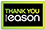# Probability and statistics for economists

Hardback
A comprehensive and up-to-date introduction to the mathematics that all economics students need to know Probability theory is the quantitative language used to handle uncertainty and is the foundation of modern statistics. Probability and Statistics for Economists provides graduate and PhD students with an essential introduction to mathematical probability and statistical theory, which are the basis of the methods used in econometrics. This incisive textbook teaches fundamental concepts, emphasizes modern, real-world applications, and gives students an intuitive understanding of the mathematics that every economist needs to know. Covers probability and statistics with mathematical rigor while emphasizing intuitive explanations that are accessible to economics students of all backgroundsDiscusses random variables, parametric and multivariate distributions, sampling, the law of large numbers, central limit theory, maximum likelihood estimation, numerical optimization, hypothesis testing, and moreFeatures hundreds of exercises that enable students to learn by doingIncludes an in-depth appendix summarizing important mathematical results as well as a wealth of real-world examplesCan serve as a core textbook for a first-semester PhD course in econometrics and as a companion book to Bruce E. Hansen's Econometrics Also an invaluable reference for researchers and practitioners
€77.00231 Reward Points
In stock online
Delivery in 2-3 working days
Free Delivery on this item

Any purchases for more than €10 are eligible for free delivery anywhere in the UK or Ireland!

A comprehensive and up-to-date introduction to the mathematics that all economics students need to know Probability theory is the quantitative language used to handle uncertainty and is the foundation of modern statistics. Probability and Statistics for Economists provides graduate and PhD students with an essential introduction to mathematical probability and statistical theory, which are the basis of the methods used in econometrics. This incisive textbook teaches fundamental concepts, emphasizes modern, real-world applications, and gives students an intuitive understanding of the mathematics that every economist needs to know. Covers probability and statistics with mathematical rigor while emphasizing intuitive explanations that are accessible to economics students of all backgroundsDiscusses random variables, parametric and multivariate distributions, sampling, the law of large numbers, central limit theory, maximum likelihood estimation, numerical optimization, hypothesis testing, and moreFeatures hundreds of exercises that enable students to learn by doingIncludes an in-depth appendix summarizing important mathematical results as well as a wealth of real-world examplesCan serve as a core textbook for a first-semester PhD course in econometrics and as a companion book to Bruce E. Hansen's Econometrics Also an invaluable reference for researchers and practitioners
Quantity:
In stock online
Delivery in 2-3 working days
Free Delivery on this item231 Reward Points

Any purchases for more than €10 are eligible for free delivery anywhere in the UK or Ireland!

€77.00
In stock online
Delivery in 2-3 working days
Free Delivery on this item
Quantity:231 Reward Points

Any purchases for more than €10 are eligible for free delivery anywhere in the UK or Ireland!